Congruency of triangles

Congruence of Triangles
Concept wise

Let suppose two triangles are congruent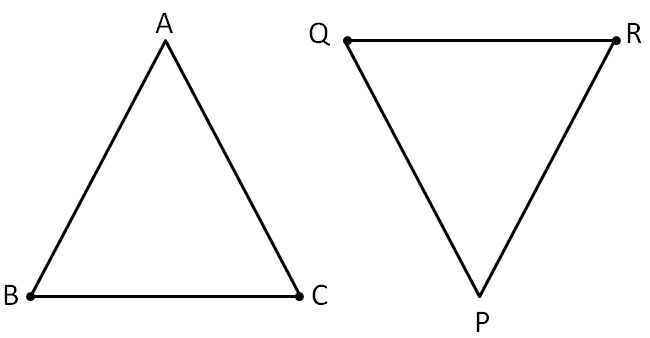We know that two triangles are congruent when

• They have same shape
• They have same size

Let’s rotate PQR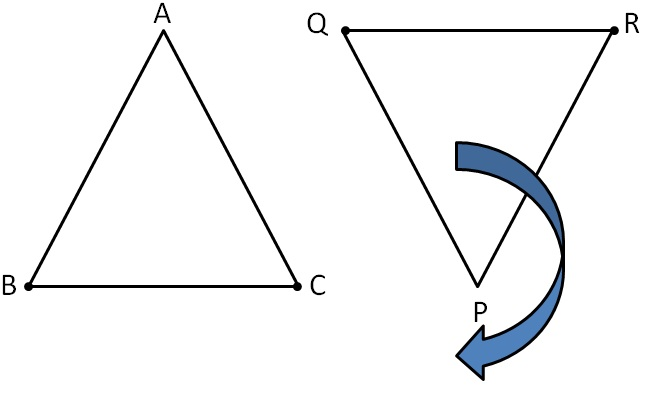And super impose ∆ABC & ∆PQR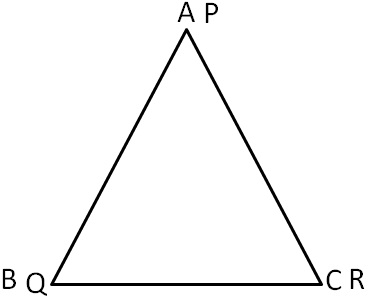So,

Points match

A ⟷ P

B ⟷ Q

C ⟷ R

Thus, we write

∆ABC ≅ ∆PQR

Not

∆ABC ≅ ∆QRP

∆ABC ≅ ∆PRQ

Because, in

∆ABC ≅ ∆PQR

A ⟷ P

B ⟷ Q

C ⟷ R

But, why is this order important?

Because of CPCT,

CPCT is corresponding parts of Congruent Triangles

If two triangles are congruent,

• Their corresponding sides are equal
• Their corresponding angles are equal

Now,

In ∆ABC & ∆PQR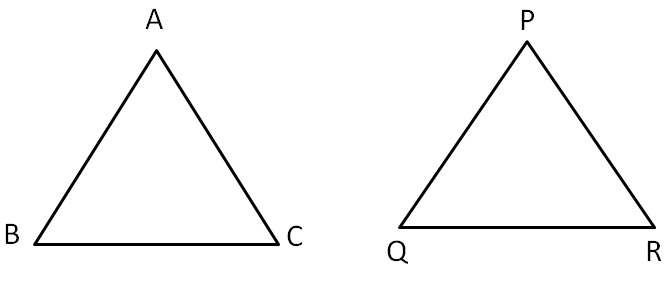If ∆ABC ≅ ∆PQR

Then,

 Corresponding angles are equal Corresponding sides are equal ∠A = ∠P AB = PQ ∠B = ∠Q BC = QR ∠C = ∠R AC = PR

Let’s check more examples

### Which triangles are congruent?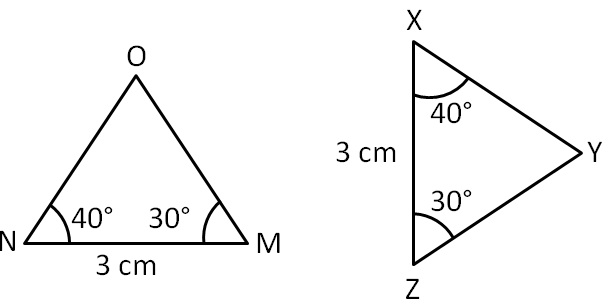Then,

 Corresponding angles are equal Corresponding sides are equal ∠M = ∠Z MN = ZX ∠N = ∠X NO = XY ∠O = ∠Y OM = YZ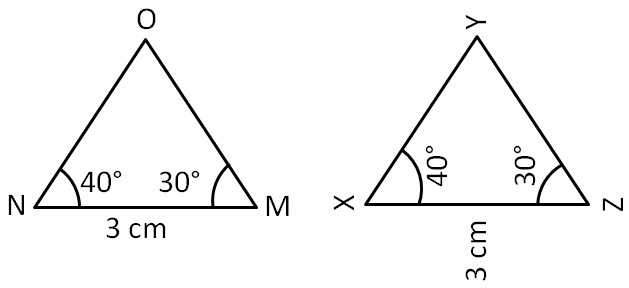Here,

M ⟷ Z

N ⟷ X

O ⟷ Y

So,

∆MNO ≅ ∆ZXY

### Which triangles are congruent?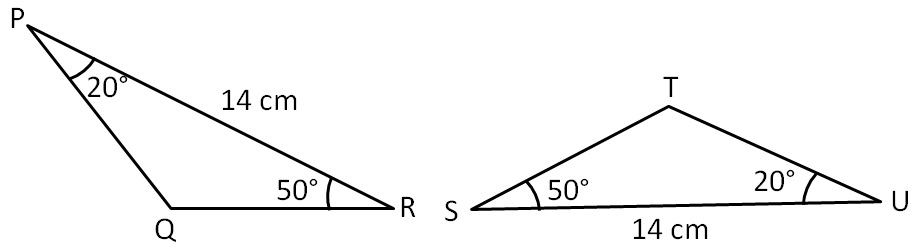Then,

 Corresponding angles are equal Corresponding sides are equal ∠P = ∠U PR = US ∠R = ∠S QR = TS ∠Q = ∠T PQ = UT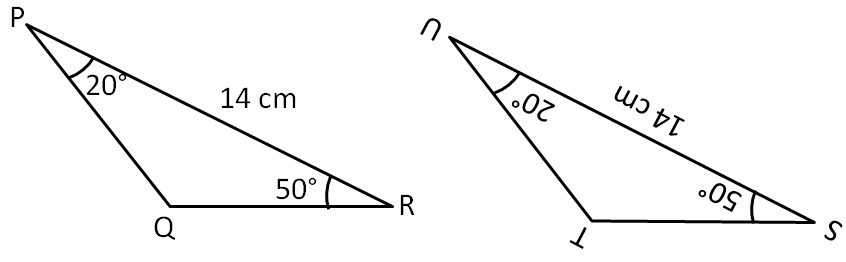Here,

P ⟷ U

R ⟷ S

Q ⟷ T

So,

∆PQR ≅ ∆UTS

### Which triangles are congruent?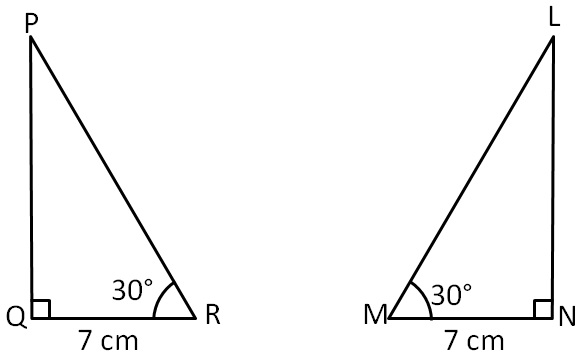Then,

 Corresponding angles are equal Corresponding sides are equal ∠P = ∠L PQ = LN ∠Q = ∠N QR = NM ∠R = ∠M PR = LM

Here,

Q ⟷ N

R ⟷ M

P ⟷ L

So,

∆PQR ≅ ∆LNM

### Which triangles are congruent?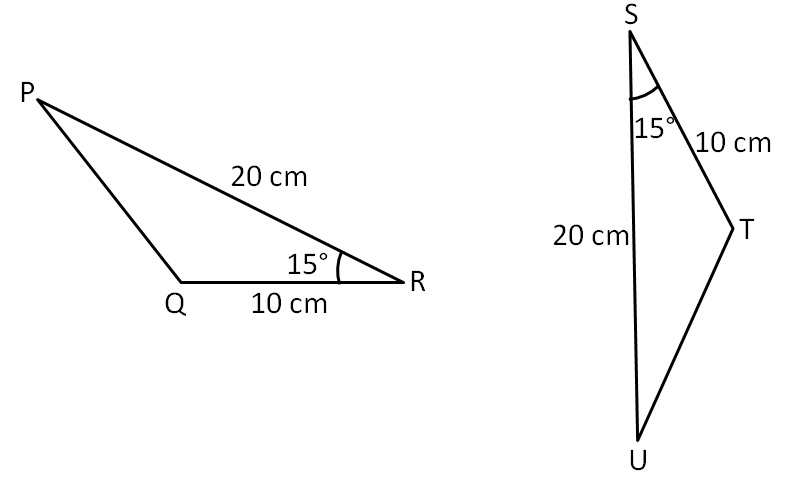Here,

R ⟷ S

P ⟷ U

Q ⟷ T

∴ ∆PQR ≅  ∆UTS

### Which triangles are congruent?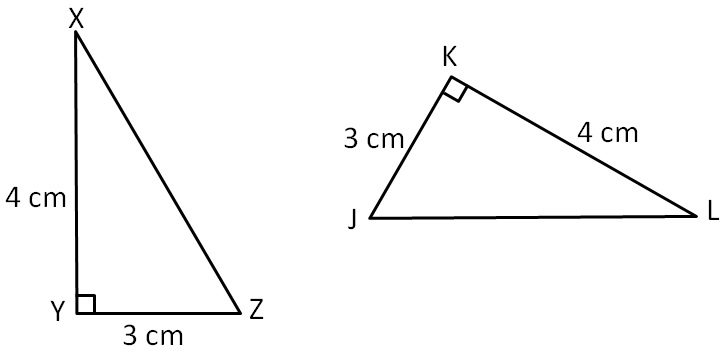Here,

Y ⟷ K

Z ⟷ J

X ⟷ L

So, ∆XYZ ≅  ∆LKJ

### Which triangles are congruent?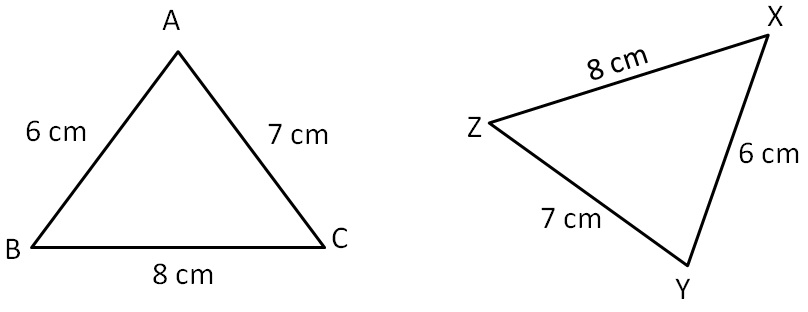Here,

A ⟷ Y

B ⟷ X

C ⟷ Z

So, ∆ABC ≅  ∆YXZ

### Which triangles are congruent?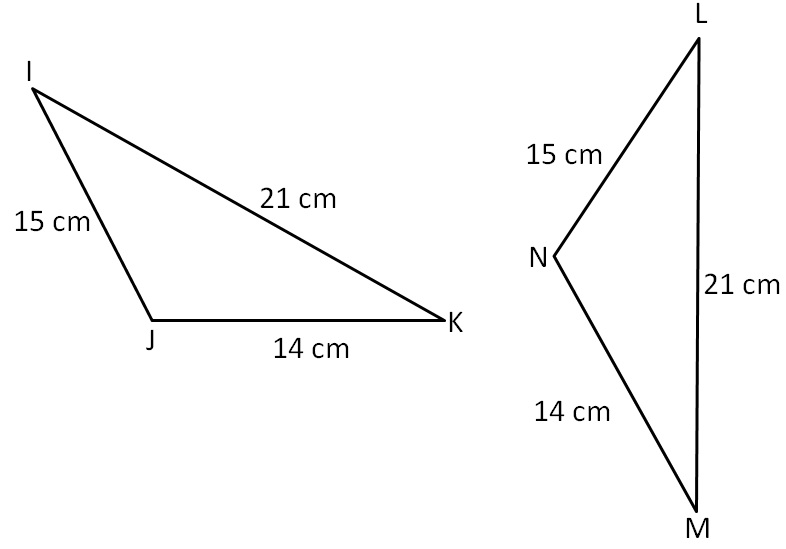Here,

J ⟷ N

I ⟷ L

K ⟷ M

So, ∆IJK ≅ ∆LNM

### Which triangles are congruent?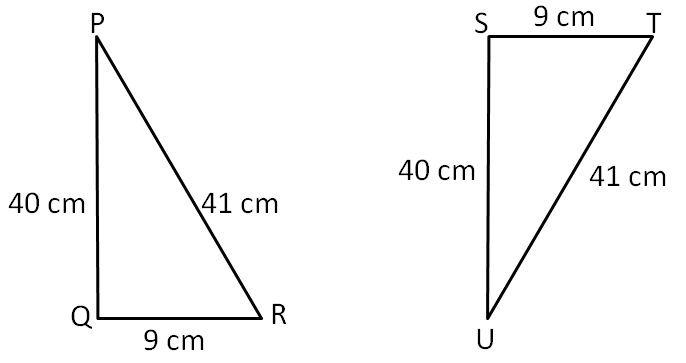Here,

P ⟷ U

Q ⟷ S

R ⟷ T

So, ∆PQR ≅ ∆UST

### Which triangles are congruent?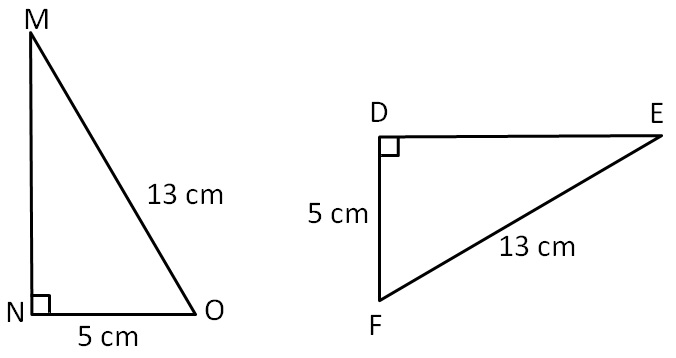Here,

N ⟷ D

O ⟷ F

M ⟷ E

So, ∆MNO ≅ ∆EDF

Learn in your speed, with individual attention - Teachoo Maths 1-on-1 Class